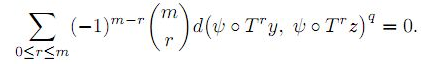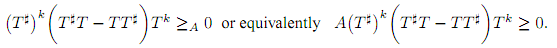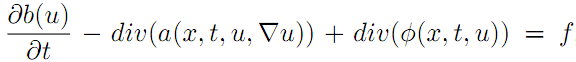The Australian Journal of Mathematical Analysis and Applications

 Home News Editors Volumes RGMIA Subscriptions Authors Contact

ISSN 1449-5910

You searched for ahmed
Total of 29 results found in site

10: Paper Source PDF document

Paper's Title:

ψ(m,q)-Isometric Mappings on Metric Spaces

Author(s):

Sid Ahmed Ould Beinane, Sidi Hamidou Jah and Sid Ahmed Ould Ahmed Mahmoud

Mathematical Analysis and Applications, Mathematics Department, College of Science,
Jouf University,
Sakaka P.O.Box 2014,
Saudi Arabia.
E-mail: beinane06@gmail.com

Department of Mathematics, College of Science Qassim University,
P.O. Box 6640, Buraydah 51452,
Saudi Arabia.
E-mail: jahsiidi@yahoo.fr

Mathematical Analysis and Applications,
Mathematics Department, College of Science, Jouf University,
Sakaka P.O.Box 2014,
Saudi Arabia.
E-mail: sidahmed@ju.edu.sa, sidahmed.sidha@gmail.com

Abstract:

The concept of (m,p)-isometric operators on Banach space was extended to (m,q)-isometric mappings on general metric spaces in . This paper is devoted to define the concept of ψ(m, q)-isometric, which is the extension of A(m, p)-isometric operators on Banach spaces introduced in . Let T,ψ: (E,d) -> (E, d) be two mappings.
For some positive integer m and q (0,). T is said to be an ψ(m,q)-isometry, if for all  y,z E,4: Paper Source PDF document

Paper's Title:

Hyponormal and K-Quasi-Hyponormal Operators On Semi-Hilbertian Spaces

Author(s):

Ould Ahmed Mahmoud Sid Ahmed and Abdelkader Benali

Mathematics Department,
College of Science,
Aljouf University,
Aljouf 2014,
Saudi Arabia.
E-mail: sididahmed@ju.edu.sa

Mathematics Department, Faculty of Science,
Hassiba Benbouali, University of Chlef,
B.P. 151 Hay Essalem, Chlef 02000,
Algeria.
E-mail: benali4848@gmail.com

Abstract:

Let H be a Hilbert space and let A be a positive bounded operator on H. The semi-inner product < u|v>A:=<Au|v>, u,v H induces a semi-norm || .||A on H. This makes H into a semi-Hilbertian space. In this paper we introduce the notions of hyponormalities and k-quasi-hyponormalities for operators on semi Hilbertian space (H,||.||A), based on the works that studied normal, isometry, unitary and partial isometries operators in these spaces. Also, we generalize some results which are already known for hyponormal and quasi-hyponormal operators. An operator T BA (H) is said to be (A, k)-quasi-hyponormal if3: Paper Source PDF document

Paper's Title:

Existence of Bounded Solutions for a Class of Strongly Nonlinear Elliptic Equations in Orlicz-Sobolev Spaces

Author(s):

Abdelmoujib Benkirane and Ahmed Youssfi

Department of Mathematics and Informatics, Faculty of Sciences Dhar El Mahraz
University Sidi Mohammed Ben Abdallah
PB 1796 Fez-Atlas, Fez
Morocco
a.benkirane@menara.ma
ahmed.youssfi@caramail.com

Abstract:

We prove, in the setting of Orlicz-Sobolev spaces, the existence of bounded solutions for some strongly nonlinear elliptic equations with operator of the principal part having degenerate coercivity and lower order terms not satisfying the sign condition. The data have a suitable summability and no Δ2-condition is needed for the considered N-functions.

2: Paper Source PDF document

Paper's Title:

Mapped Chebyshev Spectral Methods for Solving Second Kind Integral Equations on the Real Line

Author(s):

Ahmed Guechi and Azedine Rahmoune

Department of Mathematics, University of Bordj Bou Arréridj,
El Anasser, 34030, BBA,
Algeria.
E-mail: a.guechi2017@gmail.com
E-mail: a.rahmoune@univ-bba.dz

Abstract:

In this paper we investigate the utility of mappings to solve numerically an important class of integral equations on the real line. The main idea is to map the infinite interval to a finite one and use Chebyshev spectral-collocation method to solve the mapped integral equation in the finite interval. Numerical examples are presented to illustrate the accuracy of the method.

2: Paper Source PDF document

Paper's Title:

Optimization Techniques on Affine Differential Manifolds

Author(s):

Ali S Rasheed, Faik Mayah and Ahmed A H AL-Jumaili

Ministry of Higher Education and Scientific Research,
Iraq.
E-mail: ahmedhashem@gmail.com

Department of Physics, College of Sciences,
University of Wasit,
Iraq.
E-mail: faik.mayah@gmail.com

Abstract:

In addition to solid ground of Riemannian manifolds fundamentals, this article interviews some popular optimization methods on Riemannian manifolds. Several optimization problems can be better stated on manifolds rather than Euclidean space, such as interior point methods, which in turns based on self-concordant functions (logarithmic barrier functions). Optimization schemes like the steepest descent scheme, the Newton scheme, and others can be extended to Riemannian manifolds. This paper introduces some Riemannian and non-Riemannian schemes on manifolds.

2: Paper Source PDF document

Paper's Title:

Numerical Solution of Certain Types of Fredholm-Volterra Integro-Fractional Differential Equations via Bernstein Polynomials

Author(s):

Alias B. Khalaf1, Azhaar H. Sallo2 and Shazad S. Ahmed3

1Department of Mathematics, College of Science,
University of Duhok,
Kurdistan Region,
Iraq.
E-mail:  aliasbkhalaf@uod.ac

2Department of Mathematics, College of Science,
University of Duhok,
Kurdistan Region,
Iraq.
E-mail:  azhaarsallo@uod.ac

3Department of Mathematics, College of Science,
University of Sulaimani,
Kurdistan Region,
Iraq.

Abstract:

In this article we obtain a numerical solution for a certain fractional order integro-differential equations of Fredholm-Volterra type, where the fractional derivative is defined in Caputo sense. The properties of Bernstein polynomials are applied in order to convert the fractional order integro-differential equations to the solution of algebraic equations. Some numerical examples are investigated to illustrate the method. Moreover, the results obtained by this method are compared with the exact solution and with the results of some existing methods as well.

1: Paper Source PDF document

Paper's Title:

Generalized Fuglede-Putnam Theorem and Orthogonality

Author(s):

A. Bachir and A. Sagres

Department of Mathematics, Faculty of Science, King Khaled University, Abha, P.O. Box 9004 Kingdom Saudi Arabia

Abstract:

An asymmetric Fuglede-Putnam’s theorem for dominant operators and p-hyponormal operators is proved, as a consequence of this result, we obtain that the range of the generalized derivation induced by the above classes of operators is orthogonal to its kernel.

1: Paper Source PDF document

Paper's Title:

Hyers-Ulam-Rassias Stability of a Generalized Jensen Functional Equation

Author(s):

A. Charifi, B. Bouikhalene, E. Elqorachi and A. Redouani

Department of
Mathematics, Faculty of Sciences,
Ibn Tofail University,
Kenitra, Morocco
charifi2000@yahoo.fr bbouikhalene@yahoo.fr

Department of
Mathematics, Faculty of Sciences,
Ibn Zohr University,
elqorachi@hotmail.com Redouani-ahmed@yahoo.fr

Abstract:

In this paper we obtain the Hyers-Ulam-Rassias stability for the generalized Jensen's functional equation in abelian group (G,+). Furthermore we discuss the case where G is amenable and we give a note on the Hyers-Ulam-stability of the K-spherical (n × n)-matrix functional equation.

1: Paper Source PDF document

Paper's Title:

Renormalized Solutions for Nonlinear Parabolic Equation with Lower Order Terms

Author(s):

A. Aberqi1, J. Bennouna1, M. Mekkour1 and H. Redwane2

1Université Sidi Mohammed Ben Abdellah,
Département de Mathématiques,
Laboratoire LAMA, Faculté des Sciences Dhar-Mahrez,
B.P 1796 Atlas Fés,
Morocco.

2Faculté des Sciences Juridiques, Economiques et Sociales,
Université Hassan 1, B.P. 784. Settat,
Morocco.

Abstract:

In this paper, we study the existence of renormalized solutions for the nonlinear parabolic problem:,where the right side belongs to L1(Ω×(0,T)) and b(u) is unbounded function of u, the term div(a(x,t,u,u)) is a Leray--Lions operator and the function φ is a nonlinear lower order and satisfy only the growth condition.

1: Paper Source PDF document

Paper's Title:

Analysis of a Frictional Contact Problem for Viscoelastic Piezoelectric Materials

Author(s):

Meziane Said Ameur, Tedjani Hadj Ammar and Laid Maiza

Departement of Mathematics,
El Oued University,
P.O. Box 789, 39000 El Oued,
Algeria.
E-mail: said-ameur-meziane@univ-eloued.dz

Departement of Mathematics,
El Oued University,
P.O. Box 789, 39000 El Oued,
Algeria.

Department of Mathematics,
Kasdi Merbah University,
30000 Ouargla,
Algeria.
E-mail: maiza.laid@univ-ouargla.dz

Abstract:

In this paper, we consider a mathematical model that describes the quasi-static process of contact between two thermo-electro-viscoelastic bodies with damage and adhesion. The damage of the materials caused by elastic deformations. The contact is frictional and modeled with a normal compliance condition involving adhesion effect of contact surfaces. Evolution of the bonding field is described by a first order differential equation. We derive variational formulation for the model and prove an existence and uniqueness result of the weak solution. The proof is based on arguments of evolutionary variational inequalities, parabolic inequalities, differential equations, and fixed point theorem.

1: Paper Source PDF document

Paper's Title:

Analysis of a Dynamic Elasto-viscoplastic Frictionless Antiplan Contact Problem with Normal Compliance

Author(s):

A. Ourahmoun1, B. Bouderah2, T. Serrar3

1,2Applied Mathematics Laboratory,
M'sila University, 28000,
Algeria.
E-mail: ourahmounabbes@yahoo.fr

3Applied Mathematics Laboratory,
Setif 1 University, 19000,
Algeria.

Abstract:

We consider a mathematical model which describes the dynamic evolution of a thermo elasto viscoplastic contact problem between a body and a rigid foundation. The mechanical and thermal properties of the obstacle coating material near its surface. A variational formulation of this dynamic contact phenomenon is derived in the context of general models of thermo elasto viscoplastic materials. The displacements and temperatures of the bodies in contact are governed by the coupled system consisting of a variational inequality and a parabolic differential equation. The proof is based on a classical existence and uniqueness result on parabolic inequalities,differential equations and fixed point arguments.

1: Paper Source PDF document

Paper's Title:

Semivectorial Bilevel Optimization on Affine-Finsler-Metric Manifolds

Author(s):

Faik Mayah1, Ali S Rasheed2 and Naseif J. Al- Jawari3

1Department of Physics,
College of Sciences,
University of Wasit,
Iraq.
E-mail: faik.mayah@gmail.com

2Ministry of Higher Education and Scientific Research,
Iraq.
E-mail: ali.math2018@yahoo.com ahmedhashem@gmail.com

3Dept. of Mathematics,
College of Science,